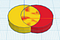# Codeblocks Fundamentals: Using the Create Group Codeblock for Addition (Union), Subtraction, and Intersection of Shapes

The addition and subtraction operations on 3D Shapes are built into Tinkercad. Both the addition and subtration operations are executed by the
Create Group codeblock. The result is a new object. Click on the Export button to export the object as a .stl file (for 3D printing). If you are unfamiliar with using Tinkercad’s Codeblocks please see: An Introduction to Tinkercad Codeblocks. We begin by executing the addition operation for 2 overlapping cylinders.

Consider the codeblocks below:

The first codeblock is for a Cylinder shape. We changed its color to red, and set its parameter values as shown. The Move codeblock moves it 8 units along the X-axis, so that it will have an intersection with a yellow cylinder that is added next.
The codeblock for the yellow cylinder is exactly like that of the green cylinder, except its color is yellow. Automatically, the yellow cylinder’s midpoint is placed on the workplane at X=0, Y=0, Z=0.

The Create Group codeblock combines (addition operation) the red and yellow cylinder into one object. The color button on the Create Group codeblock was used to set the color of this resulting object to green.

Press the Run button to see the execution of the above codeblocks. You can also watch an animation of the above via the .gif file below.

There are many examples of using the addition operation in the Codeblocks “Starters” examples of 3D designs.

The Subtraction Operation
You need a hole to do a subtraction operation. We changed the red cylinder into a hole by clicking on its gray-stripe icon.

Watch an animation of the above via a .gif file.

Intersection

The intersection of two shapes is the area that they have in common. At the present time, Tinkercad’s Codeblocks does not have a simple way to get the intersection of two shapes.

Below are the blockcodes to get the intersection of two shapes. We made the red cylinder a hole and the Move codeblock moved it so its center is at X=8, Y=0, Z=0. The codeblock for the yellow cylinder is the same as it was.

Note that the hole icon button on the multi-color Create Group codeblock was selected. This means that when the Create Group codeblock combines the red-hole cylinder with the yellow cylinder in a subtraction operation, the resulting object was turned into a hole!
This resulting hole is then combined with a yellow cylinder like the original one, in another subtraction operation. The resulting object is the intersection between the red cylinder and the yellow cylinder. The Create Group codeblock colored it green.

Watch an animation of the above via a .gif file.

Summary

We started with a red cylinder and yellow cylinder that overlapped as shown below.We started with a yellow cylinder and a red cylinder that overlapped.

The Tinkercad renderer had a hard time with the yellow-red intersection area.

Next we formed the union of the yellow cylinder and red cylinder. The Create Group codeblock was used to set the color of the object from the addition (union) operation to green.

Next we went back to what we started with above, and did a subtraction operation. The red cylinder was made to be a hole. The Create Group codeblock performed a subtraction operation with red cylinder-hole and the yellow cylinder shape.

Lastly, we presented a small number of codeblocks to show the intersection of the yellow and red cylinders. This involved doing the subtraction operation as shown above and turning the result into a hole using the Create Group codeblock . Here is what the hole looks like:

We then combined the above hole with the yellow cylinder in a subtraction operation to get the intersection shown below. The Create Group codeblock was used to make the color of the resulting object green.

The simple algorithm to show the intersection of two overlapping shapes is:

1. Turn one of the shapes into a hole.
2. Do a subtraction operation and turn the resulting object into a hole.
3. Do another subtraction operation with the hole formed in 2. above, and the shape that was not turned into a hole in 1. above.

TinyML, AI, Edge Impulse, Arduino, Raspberry Pi, Pickleball

## More from Ron Mourant

TinyML, AI, Edge Impulse, Arduino, Raspberry Pi, Pickleball